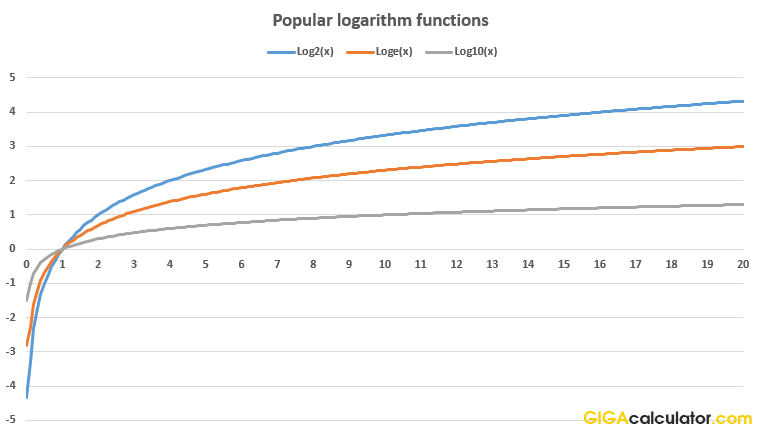# Logarithm Calculator

Use this log calculator to easily calculate the logarithm of a number with a given base: logb(x). The default base is the natural logarithm e.

Share calculator:

Embed this tool:
get code

## What is a logarithm?

The logarithm (log) operation in mathematics is the inverse to exponentiation, meaning that the log of a number is the exponent to which another fixed number called a "base" was raised to produce the number. For example log232 = 5, since 25 = 32. This is an example of a simple logarithm as it basically counts the number of multiplications of the same factor - in this case 2. The notation is logbx or logb(x) where b is the base and x is the number for which the logarithm is to be found.

There are several named logarithms: the common logarithm has a base of 10 (b = 10, log10), while the natural logarithm has a base of the number e (the Euler number, ~2.718), while the binary logarithm has a base of 2. The common logarithm has many uses in engineering, navigation, many of the sciences like physics and chemistry. The natural logarithm is widely used in math and physics due to its simpler derivative. The binary logarithm is, of course, mostly used in computer science, e.g. for representing data units. When using our logarithm calculator you need to enter a "Base" of 10 for the common logarithm, 2 for the binary logarithm, and leave the "Base" field empty to get the natural logarithm calculated.The graph above presents the values for the common, natural and binary logarithm functions for the values from 0.1 to 20 (logarithm of zero is not defined).

## How to calculate logarithms?

Certain logarithms can be easy to compute in your mind, e.g. log10(1000) = 3 since 10^3 = 1000. A general solution is to calculate logs using power series or the arithmetic-geometric mean. A pre-calculated table can also be of use if only a range of bases and logarithms are of interest on a daily basis. For anything else it is most convenient to use an online log calculator like this one due to its ease of use.

Here are some quick rules for calculating especially simple logarithms. If the base and the number are the same, e.g. log1010, the result is 1 (b1 = b for any b), while if the number is one, logb1 = 0 for any base (b0 = 1 for any b).

There are values for which the logarithm function returns negative results, e.g. log20.125 = -3, since 2-3 = 1 / 23 = 1/8 = 0.125.

## Example log calculations

log264 = 6, since 26 = 2 x 2 x 2 x 2 x 2 x 2 = 64. That's a log with base 2, log2.

log327 = 3, since 33 = 3 x 3 x 3 = 27. That's a log with base 3.

log101000 = 3, since 103 = 10 x 10 x 10 = 1000. That's a log with base 10.

## Applications of logarithms

Logarithmic scales are often used when representing wide-ranging measurements in a visually compelling graph. A prominent example is the decibel scale in which the unit (dB) expresses log-ratios of signal power and amplitude - mostly used for sound waves. pH is a well-known chemistry scale for measuring acidity. Discrete logarithms have uses in public-key cryptography, such as the one used to deliver this log calculator securely to you, making sure no one can eavesdrop on your communication with our website.

Another scale which is logarithmic is the Richter earthquake magnitude scale, measuring the earthquake's energy release. Since it is logarithmic, an earthquake of magnitude 5 is 32 times stronger (101.5) than a magnitude 4 one. A magnitude 6 earthquake releases 1,000 times (103) more energy than a magnitude 4 one.

Logarithms are used in probability theory, e.g. the law of large numbers according to which the ratio of heads to toes during tosses of a fair coin approaches 1/2 as the number of tosses approaches infinity. Some variables have log-normal distributions. The maximum likelihood estimate occurs at the same parameter-value as a maximum of the log likelihood, and the latter is easier to maximize, especially when there are multiplied likelihoods for independent random variables .

The natural logarithm is used in calculating radioactive decay and half-life of radioactive elements - the natural logarithm of 2 is used in the formulas for exponential decay. A reminder: to compute the natural log of a number in this logarithm calculator, simply leave the "Base" field empty.

Log calculations occur in fractals, entropy and chaos theory, as well as the analysis of computation complexity of different computer algorithms . They are valuable for describing algorithms in which a larger problem is divided into a set of smaller ones, and then the solutions of the smaller problems are joined to arrive at the solution of the larger problem.